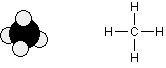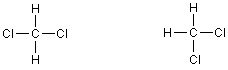# how to determine the structural formula of a molecule ??Given its molecular formula.

11 years ago

Dear mohit,

A molecular formula simply counts the numbers of each sort of atom present in the molecule, but tells you nothing about the way they are joined together.

For example, the molecular formula of butane is C4H10, and the molecular formula of ethanol is C2H6O.

Molecular formulae are very rarely used in organic chemistry, because they don't give any useful information about the bonding in the molecule.

A structural formula shows how the various atoms are bonded. There are various ways of drawing this and you will need to be familiar with all of them.

A displayed formula shows all the bonds in the molecule as individual lines. You need to remember that each line represents a pair of shared electrons.

For example, this is a model of methane together with its displayed formula:Notice that the way the methane is drawn bears no resemblance to the actual shape of the molecule. Methane isn't flat with 90° bond angles. This mismatch between what you draw and what the molecule actually looks like can lead to problems if you aren't careful.

For example, consider the simple molecule with the molecular formula CH2Cl2. You might think that there were two different ways of arranging these atoms if you drew a displayed formula.The chlorines could be opposite each other or at right angles to each other. But these two structures are actually exactly the same. Look at how they appear as models.All the best.

Sagar Singh

B.Tech IIT Delhi